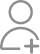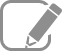Learn Vedic Maths Training from the Best Tutors

•Affordable fees
•1-1 or Group class
•Flexible Timings
•Verified Tutors

Search in

# I want to have a career in Teaching.Is Vedic Maths a better option? What is the scope of career in it?Kindly advice me about Vedic Maths and it prerequisites.Follow 11AnswerVEDIC MATHS is a fascinating subject to start with. It enhances calculation speed and reduces silly mistakes. It is useful for all( from students to job aspirants).Before starting it, you must do the proper homework. You have to design a curriculum where the different sutras have a meaningful approach...

VEDIC MATHS is a fascinating subject to start with. It enhances calculation speed and reduces silly mistakes. It is useful for all( from students to job aspirants).
Before starting it, you must do the proper homework. You have to design a curriculum where the different sutras have a meaningful approach to everyday maths. Once you are done with it, you can start and crash courses on it.
Happy teaching and happy learning!
All the very best!

It is excellent to help students with Vedic mathematics methods to create interest in Mathematics and understand the concepts better. Vedic maths will allow students to develop a sense of achievement and confidence to do tedious problems. You can take a certificate course and diploma from Kalidasa university,...

It is excellent to help students with Vedic mathematics methods to create interest in Mathematics and understand the concepts better. Vedic maths will allow students to develop a sense of achievement and confidence to do tedious problems. You can take a certificate course and diploma from Kalidasa university, Nagpur or similar universities if you are serious about it.

Vedic maths is important or not it depends upon which classes you are teaching.But it is fact that vedic maths make subject beautiful by simplifying calculation in easy way.you can learn various technique of calculation mainly in arithematic algebra...

Physics & Mathematics Expert for JEE Mains,Advanced & IMO,InMO with 9 yrs experience

I don't think using only tricks are useful for teaching purpose. Instead, learn the thought process of how these tricks were developed. For example, instead of using methods in some specific situations try to implement them in other conditions like in calculus, counting problems, etc. Eventually, the...

I don't think using only tricks are useful for teaching purpose. Instead, learn the thought process of how these tricks were developed. For example, instead of using methods in some specific situations try to implement them in other conditions like in calculus, counting problems, etc. Eventually, the student will understand all these tricks are just a workaround and don't give the actual problem-solving capabilities.

Vedic Maths is a perfect subject which allows every individual in the best way. And much faster than the regular maths which we are doing now. But, if you want to make your career in it means you have to do a lot of hard work still there is no guarantee of success. This is because of the reason that...

Vedic Maths is a perfect subject which allows every individual in the best way. And much faster than the regular maths which we are doing now.
But, if you want to make your career in it means you have to do a lot of hard work still there is no guarantee of success. This is because of the reason that the people in this generation don't have any idea and any awareness about it. And if they use these techniques in their school exams, they won't get good marks.

Professional corporate trainer with 8 years+ experience in the domain I operate in Pan India

Vedic maths helps in faster calculation through mind. There are more opportunities in schools for teaching this since it helps in students mind development.

Mathematics expert Teacher from IIT Madras - Assured Results

Vedic maths is good to learn and teach students who are aiming at Competetive exams like GMAT, CAT. Students of lower classes cany learn much except for multiplication and division. Vedic maths is not only about arithmetic. SO, I would suggest you go for conventional teaching but keep this as a hobby...

Vedic maths is good to learn and teach students who are aiming at Competetive exams like GMAT, CAT. Students of lower classes cany learn much except for multiplication and division. Vedic maths is not only about arithmetic. SO, I would suggest you go for conventional teaching but keep this as a hobby for education.A specialist, dedicated to the subject. M.Sc. in maths, Quant faculty at T.I.M.E. Mumbai.

Vedic maths is neither Vedic nor mathematics. The essence of mathematics lies in the figuring out the 'why' instead of the 'how' of any problem, and so-called Vedic maths steals it away. The name is popularized only because a sage wrote the books, which is no less than a typical 'tips and tricks' books...

Vedic maths is neither Vedic nor mathematics. The essence of mathematics lies in the figuring out the 'why' instead of the 'how' of any problem, and so-called Vedic maths steals it away. The name is popularized only because a sage wrote the books, which is no less than a typical 'tips and tricks' books in mathematics. With Sanskrit words, it just has an authentic and pure ring to it, nothing else.
So please pursue classical, perfect mathematics, and you will scale the unseen heights. All the best.

I think only Vedic Maths is not enough to start with. You should start teaching from 7-8th class in which you can add Vedic Maths subject.Good luck, Sir.

Accountancy Teacher at The Camford International School & Certified Insolvency Professional

Vedic maths helps in faster calculation through mind. There are more opportunities in schools for teaching this since it helps in students mind development.

Related Questions

Thanks to all for sparing their time and responding to my query. We have recently shifted to Noida. In case anyone knows a Vedic maths center near sector-50 where she can learn the technique it will be really helpful.
With due respect to all the vedic maths teachers and academies, the class taker shall not be under any false impression that vedic maths will help speed up calculations. Calculations can be speeded up...How will you solve the pi value? Explain please.
Kindly refer to this site :- http://www.vedicmaths.org/introduction/the-vedic-numerical-code-and-piGenius Abacus and Vedic Maths

how to be master in mathematics

As the old proverb says "Practice makes a man perfect". Becoming a Master in Mathematics is no different either. However to achieve that a good mentor plays an important role too who can give you guidance...X to the power3/5 (X to the power3/5 -7) = 8
32What is value of a & b.. in each case,

If a^b + b^a=45

If a^b X b^a=45

If a^b - b^a=45

1^44+44^1=45 So, a=1 & b=44 1^45*45^1=45 So, a=1 & b=45 46^1-1^46=45 So, a=46 & b=1Now ask question in any of the 1000+ Categories, and get Answers from Tutors and Trainers on UrbanPro.com

Related Lessons

Subtraction of a number from Base 10
Suppose subtract 358 from 10000. Here Base 1000=10'3, 10 raised to power 3 How to calculate faster in mind, just subtract 3 from 9, 5 from 9 and last digit 8 from 10. Now we get 642. Isn't it so faster...Vedic Mathematics for finding square of a number whose unit place carries 5
As we learned from my previous lesson i,e; (In case of two 2-digited numbers whose digits in 10's place are same and sum of the digits in unit places is 10 For example:- 38 and 32 (10's place digits...
N

How to find multiplication of any number by 11
Suppose multiply 45 x 11 Easy way to multiply by 11 is write first figure | ( Add first figure and last figure) | write last figure 45 x 11 = 4 | ( 4+ 5 ) | 5 = 495Vedic Mathematics for Multiplication of two 2-digited numbers
Vedic Mathematics is a very powerful tool for us to get quick calculations For Multiplication of two 2-digited numbers, we can follow FOIL technique. If you want to multiply 74×36(let's present...
N

Vedic Math Division: Case 5
524 6 8 5 1 :2 3 5 6 0 3 3 2 6 8 -5 -2 -29 -10 -25 3 33 2 12 38 1 0 5 0 1 5 ...Looking for Vedic Maths Training classes?

Learn from the Best Tutors on UrbanPro

Are you a Tutor or Training Institute?

Join UrbanPro Today to find students near you
X

### Looking for Vedic Maths Training Classes?

The best tutors for Vedic Maths Training Classes are on UrbanPro

• Select the best Tutor
• Book & Attend a Free Demo
• Pay and start Learning### Learn Vedic Maths Training with the Best Tutors

The best Tutors for Vedic Maths Training Classes are on UrbanPro